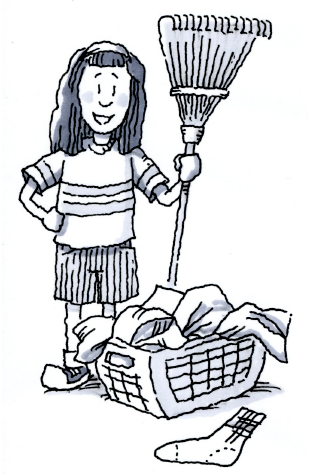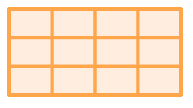### Home > CC2MN > Chapter 2 > Lesson 2.2.3 > Problem2-66

2-66.

On Saturday, Stella worked for two hours helping her mother around the house. She spent $\frac { 1 } { 3 }$ of her time doing laundry, $\frac { 1 } { 4 }$ of her time cleaning, and the rest of her time working in the yard. How much of her time was spent in the yard? Show all of your work. Use a diagram if it helps.Draw a rectangle like the one the right. A horizontal rectangle.
How can you subdivide it so you can represent one third and one fourth?
Try this before moving on.

One way to show both fractions is with a diagram: The rectangle divided into 12 equal parts with 3 rows of 4 columns

Copy this diagram and shade $\frac{1}{3}$ and $\frac{1}{4}$.

What is left?

The answer is $\frac{5}{12}$ or $50$ minutes.

How can you arrive at this answer without drawing a diagram?